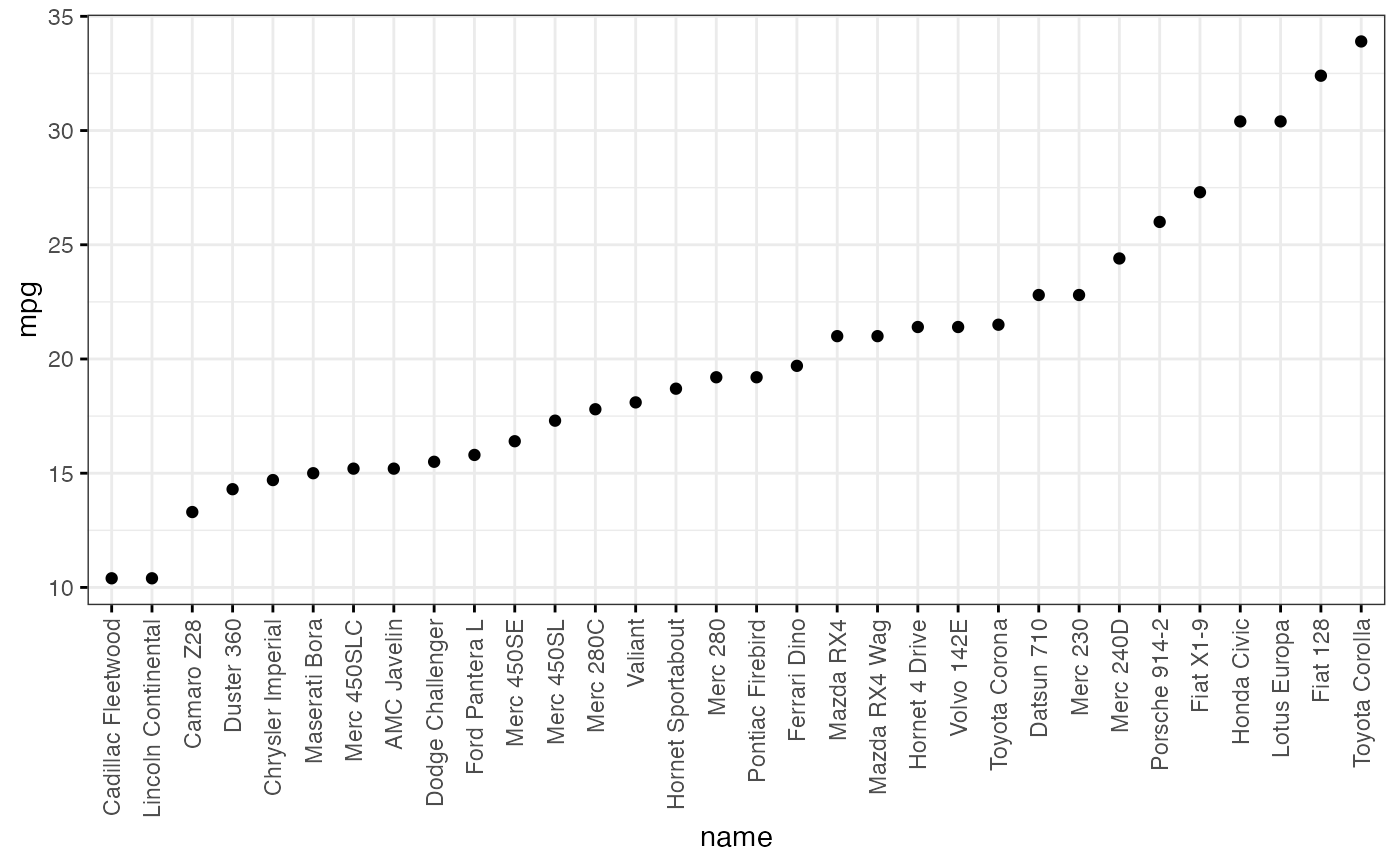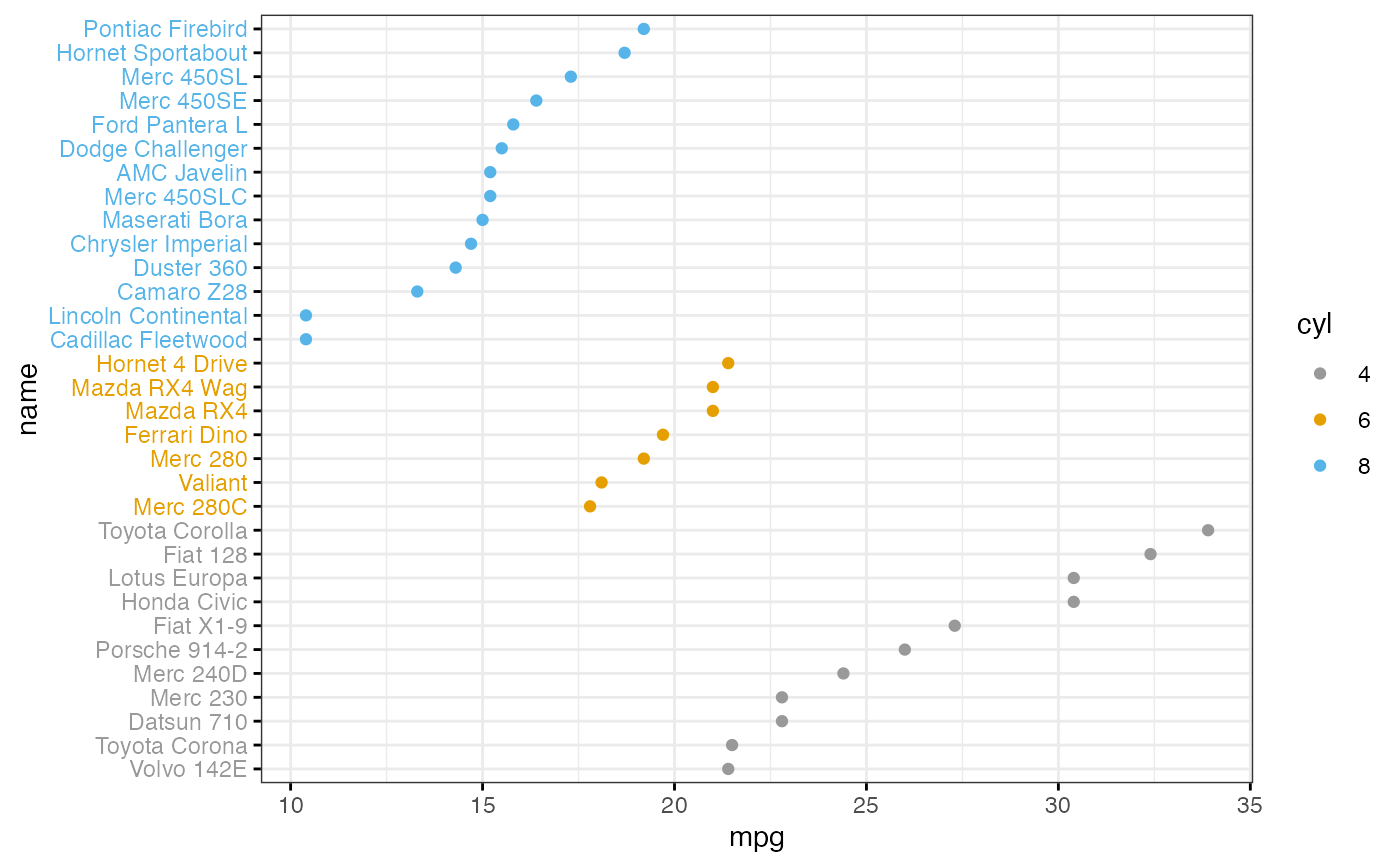Draw a Cleveland dot plot.

ggdotchart(
data,
x,
y,
group = NULL,
combine = FALSE,
color = "black",
palette = NULL,
shape = 19,
size = NULL,
dot.size = size,
sorting = c("ascending", "descending", "none"),
x.text.col = TRUE,
rotate = FALSE,
title = NULL,
xlab = NULL,
ylab = NULL,
facet.by = NULL,
panel.labs = NULL,
short.panel.labs = TRUE,
select = NULL,
remove = NULL,
order = NULL,
label = NULL,
font.label = list(size = 11, color = "black"),
label.select = NULL,
repel = FALSE,
label.rectangle = FALSE,
position = "identity",
ggtheme = theme_pubr(),
...
)

theme_cleveland(rotate = TRUE)

## Arguments

data

a data frame

x, y

x and y variables for drawing.

group

an optional column name indicating how the elements of x are grouped.

combine

logical value. Default is FALSE. Used only when y is a vector containing multiple variables to plot. If TRUE, create a multi-panel plot by combining the plot of y variables.

color, size

points color and size.

palette

the color palette to be used for coloring or filling by groups. Allowed values include "grey" for grey color palettes; brewer palettes e.g. "RdBu", "Blues", ...; or custom color palette e.g. c("blue", "red"); and scientific journal palettes from ggsci R package, e.g.: "npg", "aaas", "lancet", "jco", "ucscgb", "uchicago", "simpsons" and "rickandmorty".

shape

point shape. See show_point_shapes.

dot.size

numeric value specifying the dot size.

sorting

a character vector for sorting into ascending or descending order. Allowed values are one of "descending", "ascending" and "none". Partial match are allowed (e.g. sorting = "desc" or "asc"). Default is "descending".

character vector for adding another plot element (e.g.: dot plot or error bars). Allowed values are one or the combination of: "none", "dotplot", "jitter", "boxplot", "point", "mean", "mean_se", "mean_sd", "mean_ci", "mean_range", "median", "median_iqr", "median_hilow", "median_q1q3", "median_mad", "median_range"; see ?desc_statby for more details.

parameters (color, shape, size, fill, linetype) for the argument 'add'; e.g.: add.params = list(color = "red").

x.text.col

logical. If TRUE (default), x axis texts are colored by groups.

rotate

logical value. If TRUE, rotate the graph by setting the plot orientation to horizontal.

title

plot main title.

xlab

character vector specifying x axis labels. Use xlab = FALSE to hide xlab.

ylab

character vector specifying y axis labels. Use ylab = FALSE to hide ylab.

facet.by

character vector, of length 1 or 2, specifying grouping variables for faceting the plot into multiple panels. Should be in the data.

panel.labs

a list of one or two character vectors to modify facet panel labels. For example, panel.labs = list(sex = c("Male", "Female")) specifies the labels for the "sex" variable. For two grouping variables, you can use for example panel.labs = list(sex = c("Male", "Female"), rx = c("Obs", "Lev", "Lev2") ).

short.panel.labs

logical value. Default is TRUE. If TRUE, create short labels for panels by omitting variable names; in other words panels will be labelled only by variable grouping levels.

select

character vector specifying which items to display.

remove

character vector specifying which items to remove from the plot.

order

character vector specifying the order of items.

label

the name of the column containing point labels.

font.label

a list which can contain the combination of the following elements: the size (e.g.: 14), the style (e.g.: "plain", "bold", "italic", "bold.italic") and the color (e.g.: "red") of labels. For example font.label = list(size = 14, face = "bold", color ="red"). To specify only the size and the style, use font.label = list(size = 14, face = "plain").

label.select

can be of two formats:

• a character vector specifying some labels to show.

• a list containing one or the combination of the following components:

• top.up and top.down: to display the labels of the top up/down points. For example, label.select = list(top.up = 10, top.down = 4).

• criteria: to filter, for example, by x and y variabes values, use this: label.select = list(criteria = "y > 2 & y < 5 & x %in% c('A', 'B')").

repel

a logical value, whether to use ggrepel to avoid overplotting text labels or not.

label.rectangle

logical value. If TRUE, add rectangle underneath the text, making it easier to read.

position

Position adjustment, either as a string, or the result of a call to a position adjustment function.

ggtheme

function, ggplot2 theme name. Default value is theme_pubr(). Allowed values include ggplot2 official themes: theme_gray(), theme_bw(), theme_minimal(), theme_classic(), theme_void(), ....

...

other arguments to be passed to geom_point and ggpar.

## Details

The plot can be easily customized using the function ggpar(). Read ?ggpar for changing:

• main title and axis labels: main, xlab, ylab

• axis limits: xlim, ylim (e.g.: ylim = c(0, 30))

• axis scales: xscale, yscale (e.g.: yscale = "log2")

• color palettes: palette = "Dark2" or palette = c("gray", "blue", "red")

• legend title, labels and position: legend = "right"

• plot orientation : orientation = c("vertical", "horizontal", "reverse")

ggpar

## Examples

# Load data
data("mtcars")
df <- mtcars
df$cyl <- as.factor(df$cyl)
df\$name <- rownames(df)
#>                  wt  mpg cyl
#> Mazda RX4     2.620 21.0   6
#> Mazda RX4 Wag 2.875 21.0   6
#> Datsun 710    2.320 22.8   4

# Basic plot
ggdotchart(df, x = "name", y ="mpg",
ggtheme = theme_bw())# Change colors by  group cyl
ggdotchart(df, x = "name", y = "mpg",
group = "cyl", color = "cyl",
palette = c('#999999','#E69F00','#56B4E9'),
rotate = TRUE,
sorting = "descending",
ggtheme = theme_bw(),
y.text.col = TRUE )
#> Warning: Vectorized input to element_text() is not officially supported.
#> ℹ Results may be unexpected or may change in future versions of ggplot2.# Plot with multiple groups
# +++++++++++++++++++++
# Create some data
df2 <- data.frame(supp=rep(c("VC", "OJ"), each=3),
dose=rep(c("D0.5", "D1", "D2"),2),
len=c(6.8, 15, 33, 4.2, 10, 29.5))
print(df2)
#>   supp dose  len
#> 1   VC D0.5  6.8
#> 2   VC   D1 15.0
#> 3   VC   D2 33.0
#> 4   OJ D0.5  4.2
#> 5   OJ   D1 10.0
#> 6   OJ   D2 29.5

ggdotchart(df2, x = "dose", y = "len",
color = "supp", size = 3,